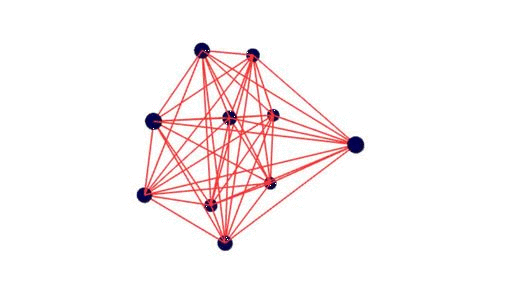dummy-link

19

8

2

2

# NetworkViz

A Julia module to render graphs in 3D using ThreeJS tightly coupled with LightGraphs.

## Install

In a Julia REPL, run:

``````Pkg.add("NetworkViz")
``````

## Graph Primitives

### NodeProperty

The `NodeProperty` type stores the properties of each node in the graph. It stores the following properties :

• `color` : It is a `Colors` array that stores the colors of all the nodes in the graph.
• `size` : Size of the node. eg : `0.2`.
• `shape` : Shape of the node. Can be 0 or 1. `0 - Square`, `1 - Circle`.

### EdgeProperty

The `EdgeProperty` type stores the properties of each edge in the graph. It stores the following properties :

• `color` : It is a hex string that stores the color of the edges.
• `width` : Thickness of the edges. eg : `1.5`.

## Visualizing Graphs

The `drawGraph` function can be used to draw the graphs in 2D or 3D with nodes having different colors. It can accept `LightGraphs.Graph` and `LightGraphs.Digraph` types. `drawGraph` can be used to draw graphs from adjacency matrices also. The function accepts an additional kwargs `node::NodeProperty`, `edge::EdgeProperty`, and `z`. If `z=1`, it draws a 3D graph. If `z=0`, a 2D visualization of the graph is drawn. `node` and `edge` determines the properties of nodes and edges respectively.

Usage :

``````g = CompleteGraph(10)
c = Color[parse(Colorant,"#00004d") for i in 1:nv(g)]
n = NodeProperty(c,0.2,0)
e = EdgeProperty("#ff3333",1)
drawGraph(g,node=n,edge=e,z=1) #Draw using a Graph object (3D).

am = full(adjacency_matrix(g))
drawGraph(am,node=n,edge=e,z=0) #Draw using an adjacency matrix (2D).

dgraph = bfs_tree(g,1)
drawGraph(dgraph,z=1) #Draw a Digraph.
``````

## Utility Functions

• `addEdge(g::Graph,node1::Int,node2::Int,z=1)` - Add a new edge `node1-node2` and redraws the graph. `z` toggles 2D-3D conversion. Fails silently if an already existing node is added again.
• `removeEdge(g::Graph,node1::Int,node2::Int,z=1)` - Removes the edge `node1-node2` if it exists and redraws the graph. `z` toggles 2D-3D conversion.
• `addNode(g::Graph,z=1)` - Adds a new node to the graph. `z` toggles 2D-3D conversion.
• `removeNode(g::Graph,node::Int,z=1)` - Removes `node` if it exists and redraws the graph. `z` toggles 2D-3D conversion.

## Examples

``````#Run this code in Escher
using NetworkViz
using LightGraphs
main(window) = begin
push!(window.assets, "widgets")
push!(window.assets,("ThreeJS","threejs"))
g = CompleteGraph(10)
drawGraph(g)
end
``````

The above code produces the following output :Here is another example with a code-mirror where functions can be typed in. Depending on the LightGraphs function used, 2D as well as 3D graphs are drawn. You can see the working demo here.

You can find many other examples in the `examples/` folder.

## Acknowledgement

IainNZ for the original Spring-Embedder code. (Taken from GraphLayout.jl).

03/04/2016

5 months ago

72 commits